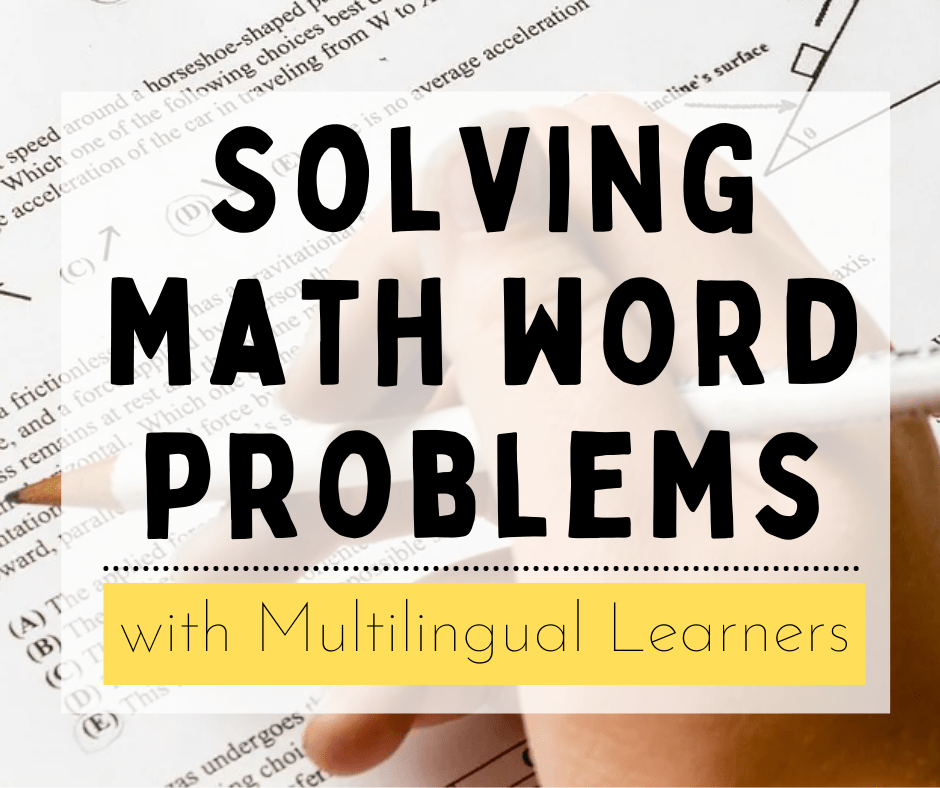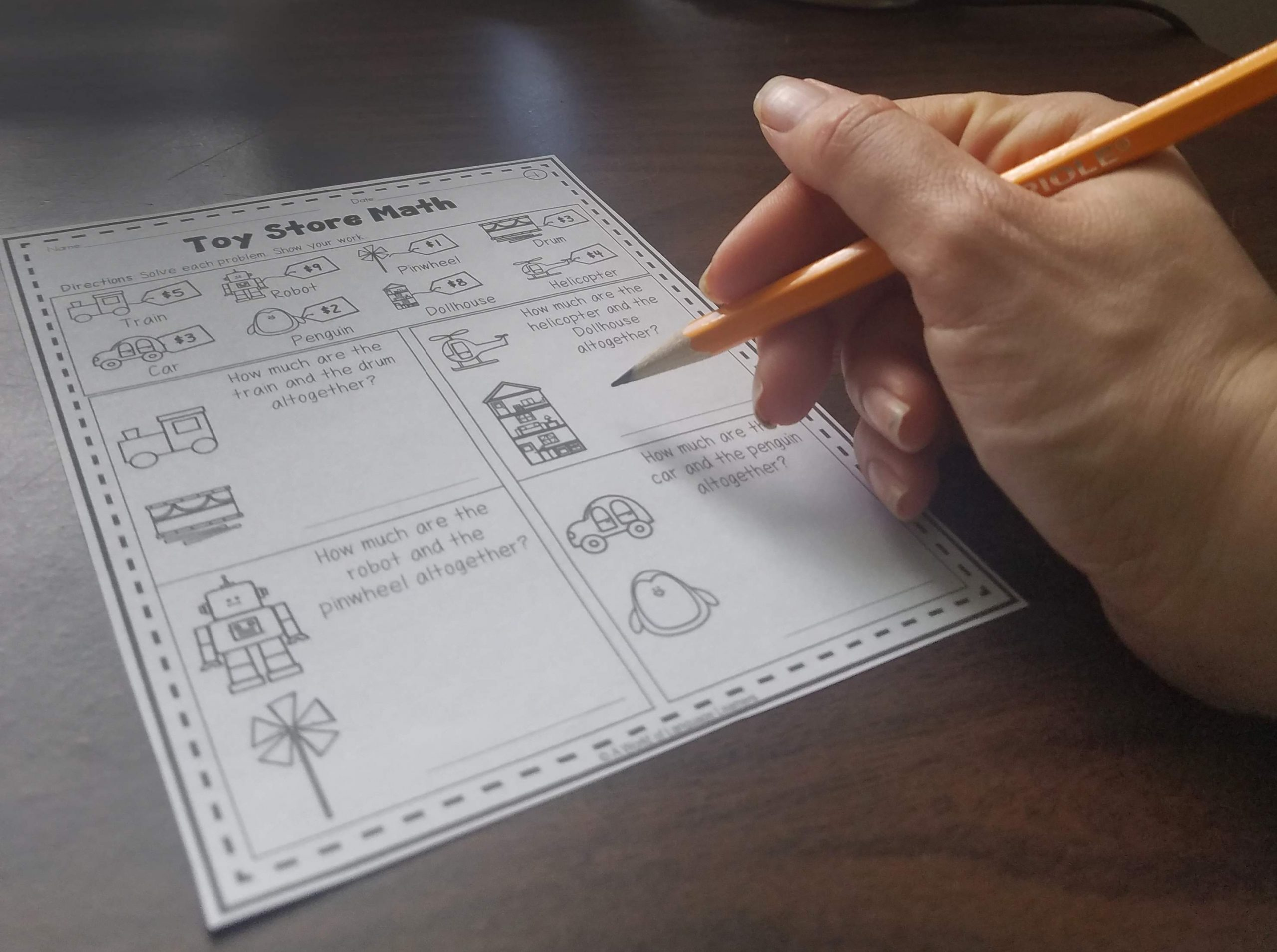Solving Math Word Problems with MLs

# Solving Math Word Problems with MLsYou may have heard that math is a universal language. This is not the case when students are solving word problems. Many students struggle to solve word problems, they can be particularly challenging for ELLs. Here are some strategies for solving math word problems with English Language Learners.

Teach students the important vocabulary for the type of word problems that they are solving. This will help students to begin to figure out that how many more might mean to add and take away often means to subtract. One caution is not to only rely on only teaching keywords to help students solve word problems.

## Draw Pictures

Have students illustrate what is happening in a word problem. This can help them to pick out the important information. A picture can also help students decide which operation to use by thinking about if the quantity is increasing or decreasing.

## Analyze the Word Problem

Help students answer questions before they try and solve the word problem. Some examples are: What do you know? What do you have to find out? The book Supporting English Language Learners in Math Class has additional examples of questions to ask ELLs as they try and solve a word problem.

## Guided Practice

One of the best ways to support ELLs with solving math word problems is to model an example word problem and then have students solve a similar problem on their own. This gives students the chance to work through a problem with support.

## Use Sentence Frames for Reflection

Provide students with sentence frames to help them reflect on how they solve a math word problem.

________ is the answer because __________.

The answer is __________. These are the steps that I took to solve the problem. First ____Then___Last_____.

## Teaching Materials

Here is a set of math word problems that uses simple language and pictures, to give students practice answering addition and subtraction word problems.Kindergarten and First Grade Math Bundles– These sets include simple word problems that follow similar sentence patterns and only the numbers change.

## Resources

Strategies for solving math word problems for ELLs from Colorin Colorado.

Supporting ELLs in Math Class– This set of books gives teachers language to use with ELLs, strategies, and examples of supporting ELLs in math class.